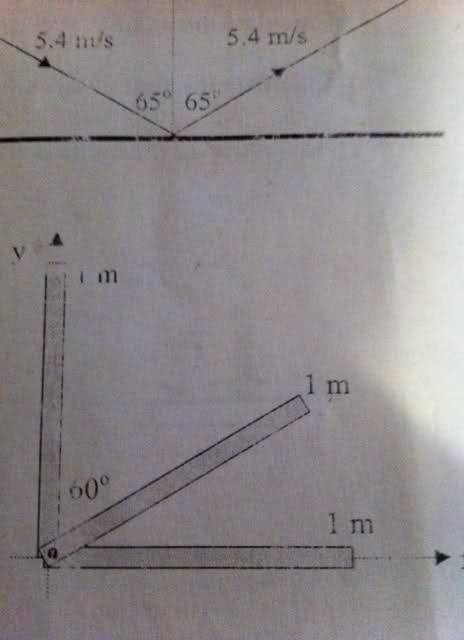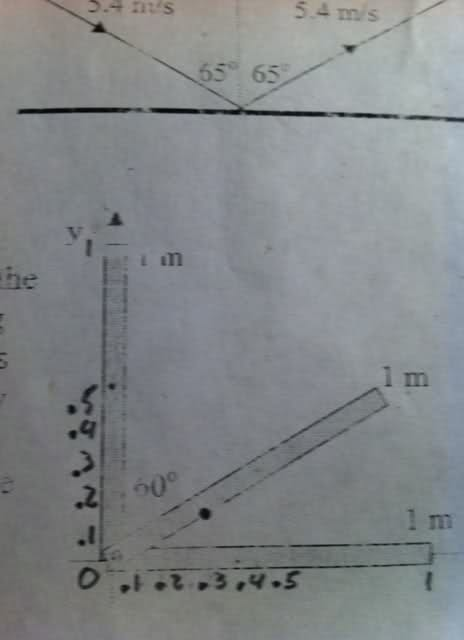# Simple center of mass question - Thanks

• nukeman

## Homework Statement

Hey all. Below is the question and picture related to it.

"Three uniform metre sticks each of mass M are attached together as follows: Stick 1 lies along the y-axis from y = 0 to y = 1.0m. Stick 2 lies along the x-axis from x = 0 to x = 1.0m. Stick 3 makes an angle of 60 degrees with the y axis. Find the x and Y coordinates of the centre of mass of the system of three metre sticks, and marks its approximate location on the diagram of the three sticks."

----Sorry, its kinda upside down :)## The Attempt at a Solution

I am really confused with this one.

The formula I know is: Xcm = m1x1 + m2x2 + m3x3 / m1+m2+m3

But how do I input into this formula... ??

Replace every metre stick with a point mass of M at its own centre of mass.

ehild

How do I find each metre sticks own center of mass??

assuming each stick is of uniform density, it will be in the physical center of the stick.

$x_{c} = \frac{\sum_{i = 1}^{N} m_{i} x_{i}}{\sum_{i = 1}^{N} m_{i}}$

$y_{c} = \frac{\sum_{i = 1}^{N} m_{i} y_{i}}{\sum_{i = 1}^{N} m_{i}}$

these describe the center of mass, but assuming it is uniform, the masses are taken out and you are left with just the physical center:

$x_{c} = \frac{\sum_{i=1}^{N} x_{i}}{N}$

$y_{c} = \frac{\sum_{i=1}^{N} y_{i}}{N}$

now plug that into the same equation for all three sticks:

$x_{cm} = \frac{x_{cm1} + x_{cm2} + x_{sm3}}{3}$

$y_{cm} = \frac{y_{cm1} + y_{cm2} + y_{sm3}}{3}$

Last edited:
assuming each stick is of uniform density, it will be in the physical center of the stick.

$x_{c} = \frac{\sum_{i = 1}^{N} m_{i} x_{i}}{\sum_{i = 1}^{N} m_{i}}$

$y_{c} = \frac{\sum_{i = 1}^{N} m_{i} y_{i}}{\sum_{i = 1}^{N} m_{i}}$

these describe the center of mass, but assuming it is uniform, the masses are taken out and you are left with just the physical center:

$x_{c} = \frac{\sum_{i=1}^{N} x_{i}}{N}$

$y_{c} = \frac{\sum_{i=1}^{N} y_{i}}{N}$

now plug that into the same equation for all three sticks:

$x_{cm} = \frac{x_{cm1} + x_{cm2} + x_{sm3}}{3}$

$y_{cm} = \frac{y_{cm1} + y_{cm2} + y_{sm3}}{3}$

What about the stick at the 60 degree angle?

Im actually having trouble with plugging the right info into the formula, let's say for X - what would let's say "Xcm1" be?

You can resolve the center of mass of the angled stick into its components using trig ratios.

$x = \frac{1}{2} \cos (\frac{\pi}{6})$

$y = \frac{1}{2} \sin (\frac{\pi}{6})$

and then proceed from there.

by $x_{cm1}$ i mean the x component of the center of mass for stick one.

is Xcm1 = .5 m?

is Xcm1 = .5 m?

well that depends on what you choose to be your first stick. if you're talking about the bottom one then you'd be correct

Ahhg, I am not getting this. :(

Thanks for trying tho

keep at it, just try to understand what the formulas are saying.

keep trying, but you can check it against this:

$x_{cm} = \frac{0 + \frac{1}{2}\cos(\frac{\pi}{6}) + 0.5}{3}$

$y_{cm} = \frac{0.5 + \frac{1}{2}\sin(\frac{\pi}{6}) + 0}{3}$

Gordon or anyone, here is what I got. Can you tell me if the answer I got to this question is correct, including image (posted below)

(.333 i + .168 j) m

Image of where centre of mass roughly would be?Last edited:
Can anyone confirm this for me please ? :)

Two words my friend, radian mode.

dammit :)